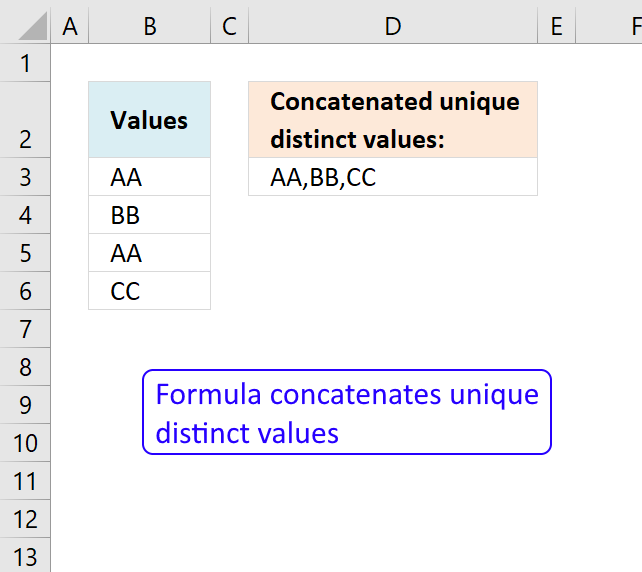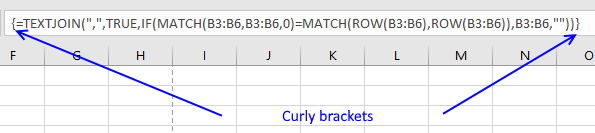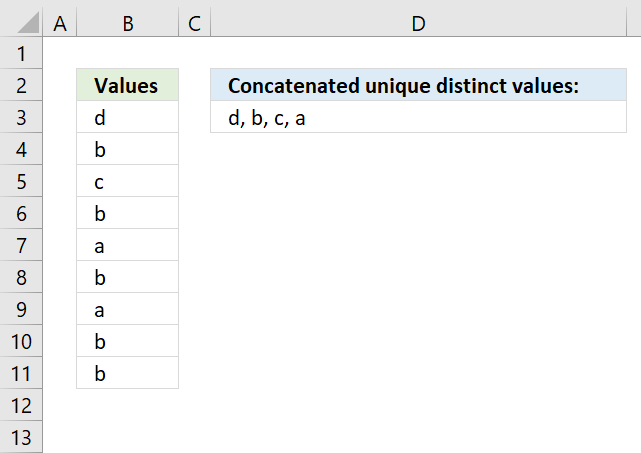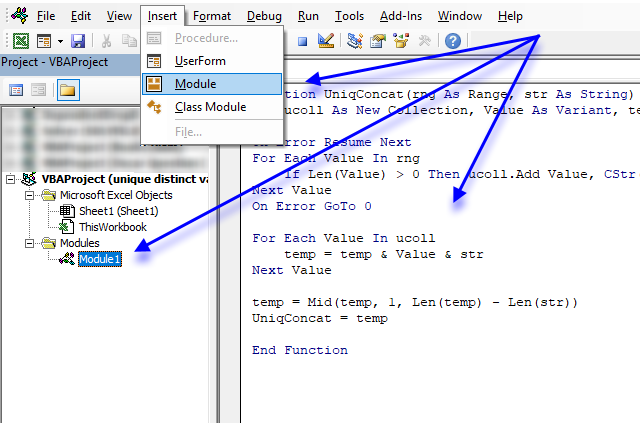Author: Oscar Cronquist Article last updated on May 23, 2019

The new TEXTJOIN function in excel 2016 lets you do some amazing things with arrays. This post demonstrates how to extract unique distinct values from a single column and concatenate the result into one cell.

The following picture shows you 4 values in column B. Value "AA" has a duplicate in cell B5. Unique distinct values are all values except duplicates.Array formula in cell D3:

=TEXTJOIN(", ", TRUE, IF(MATCH(B3:B6, B3:B6, 0)=MATCH(ROW(B3:B6), ROW(B3:B6)), B3:B6, ""))

Tip! You can use the UDF I made if you don't have Excel 2016 and the TEXTJOIN function.

Recommended article

Concatenate cell values

Joining multiple cell values in Excel is not easy, for example, the CONCATENATE function allows you to only reference a […]

#### How to enter an array formula

1. Double click on cell D3
2. Copy/Paste above formula to cell D3
3. Press and hold CTRL + SHIFT
4. Press Enter once
5. Release all keys

Your formula has now a beginning and ending curly bracket, like this:Don't enter these characters yourself, they appear automatically if you followed above steps.

Recommended article

How to enter an array formula

Array formulas allows you to do advanced calculations not possible with regular formulas.

#### Explaining array formula in cell D3

Step 1 - Match values with themselves

MATCH(B3:B6, B3:B6, 0)

becomes

MATCH({"AA"; "BB"; "AA"; "CC"}, {"AA"; "BB"; "AA"; "CC"},0)

and returns {1;2;1;4}

The MATCH function returns the relative position of a value in an array. It only returns the position of the first found value.

Value "AA" is found on the first position, so the first value in the array is 1. {1;2;1;4}

Value "BB" is found on the second position, so the second value in the array is 2. {1;2;1;4}

Value "AA" is found on the first position, so the third value in the array is 1. {1;2;1;4}

Value "CC" is found on the fourth position, so the fourth value in the array is 4. {1;2;1;4}

Recommended article

How to use the MATCH function

Identify the position of a value in an array.

Step 2 - Compare array with relative positions

MATCH(B3:B6, B3:B6, 0)=MATCH(ROW(B3:B6), ROW(B3:B6))

becomes

{1;2;1;4}={1;2;3;4}

and returns {TRUE;TRUE;FALSE;TRUE}

This array tells us if a value is a duplicate or not. FALSE indicates a duplicate and we don't want that in the final result.

Step 3 - Filter unique distinct values

IF(MATCH(B3:B6, B3:B6, 0)=MATCH(ROW(B3:B6), ROW(B3:B6)), B3:B6, "")

becomes

IF({TRUE;TRUE;FALSE;TRUE}, B3:B6, "")

becomes

IF({TRUE;TRUE;FALSE;TRUE}, {"AA";"BB";"AA";"CC"}, "")

and returns {"AA";"BB";"";"CC"}

Recommended article

How to use the IF function

Checks if a logical expression is met. Returns a specific value if TRUE and another specific value if FALSE.

Step 4 - Concatenate values

TEXTJOIN(", ", TRUE, IF(MATCH(B3:B6, B3:B6, 0)=MATCH(ROW(B3:B6), ROW(B3:B6)), B3:B6, ""))

becomes

TEXTJOIN(", ", TRUE, {"AA";"BB";"";"CC"})

and returns AA,BB,CC.

The first argument in the TEXTJOIN function lets you specify the delimiting character. The second argument if you want to ignore empty strings. The third argument is the values you want to concatenate.

Recommended article

How to use the TEXTJOIN function

The TEXTJOIN function is a new function for office 365 subscribers, it is like the CONCATENATE function on steroids or what the CONCATENATE function […]

Make sure you check out these links if you want to know more about the excel functions in the array formula above: TEXTJOIN, MATCH, IF, ROW

Concatenate unique distinct values.xlsx

### Concatenate unique distinct values (VBA)The formula in cell D3 contains a User defined Function that extracts unique distinct values concatenated, you have the option to specify the delimiting character in the second argument.

=UniqConcat(B3:B11,", ")

The formula is a regular formula, however, you need to copy the code below and paste it to a code module in your workbook before you use it.

#### VBA code

```Function UniqConcat(rng As Range, str As String)
Dim ucoll As New Collection, Value As Variant, temp As String

On Error Resume Next
For Each Value In rng
If Len(Value) > 0 Then ucoll.Add Value, CStr(Value)
Next Value
On Error GoTo 0

For Each Value In ucoll
temp = temp & Value & str
Next Value

temp = Mid(temp, 1, Len(temp) - Len(str))
UniqConcat = temp

End Function

```

#### Where to put the code?1. Press Alt + F11 to open the VB Editor.
2. Click on "Insert" on the menu.
3. Click on "Module".
4. Copy above code.
5. Paste to code module.